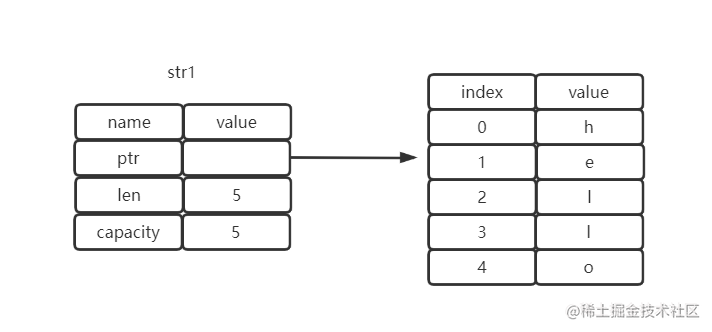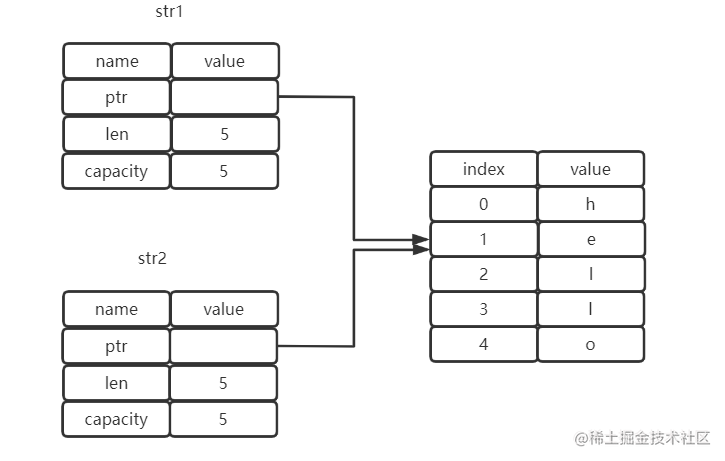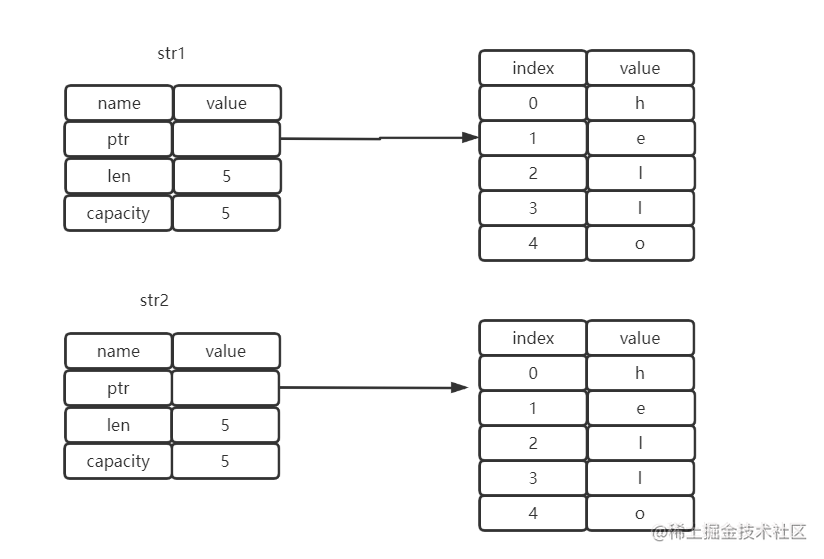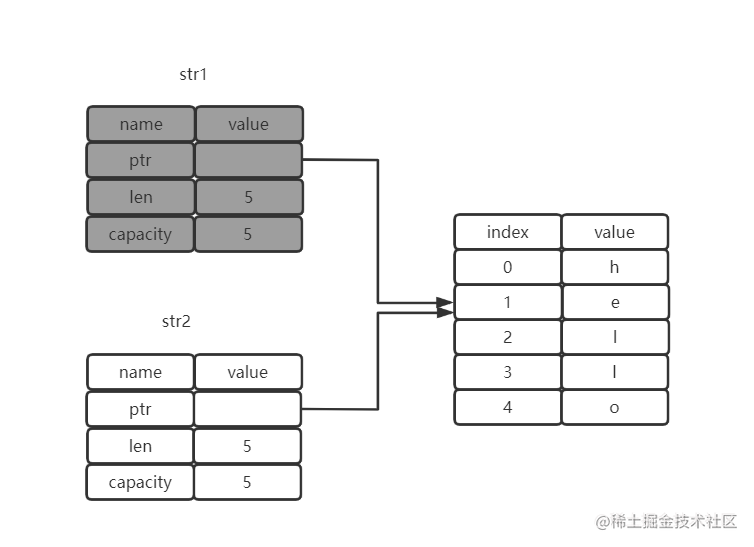# 变量与数据的交互方式 - 移动

Rust 中的多个变量可以采用一种比较独特的方式和同一个数据进行交互，如下代码所示，将变量`x`的值赋给`y`

``````fn main() {
let x = 1;
let y = x;
}

``````fn main() {
let str1 = String::from("hello");
let str2 = str1;
}``````fn main() {
let s1 = String::from("hello");
let s2 = s1;

println!("{}, world!", s1);
}

``````error[E0382]: use of moved value: `s1`
--> src/main.rs:5:28
|
3 |     let s2 = s1;
|         -- value moved here
4 |
5 |     println!("{}, world!", s1);
|                            ^^ value used here after move
|
= note: move occurs because `s1` has type `std::string::String`, which does
not implement the `Copy` trait